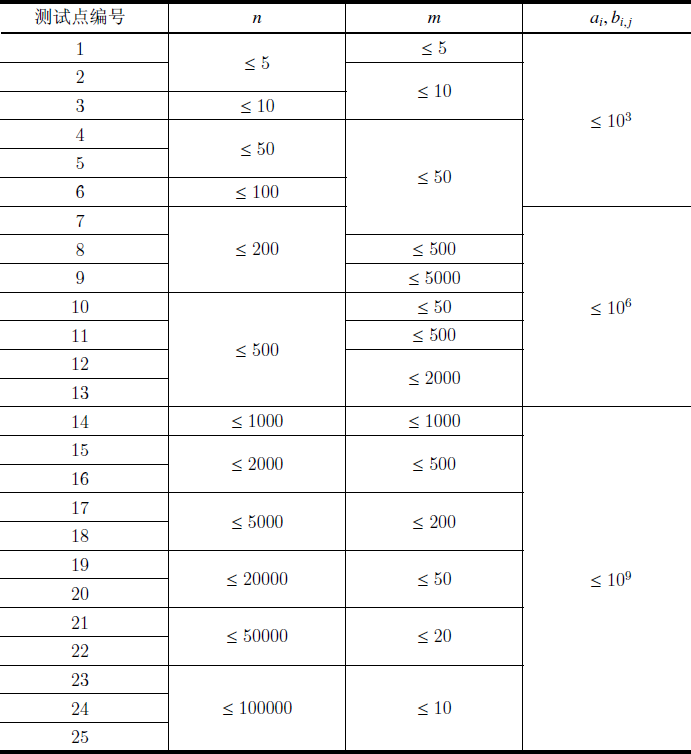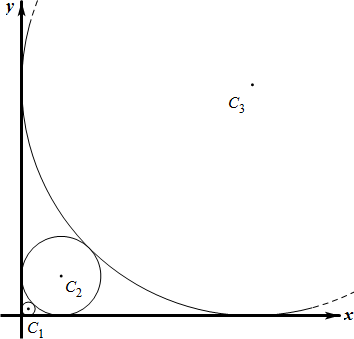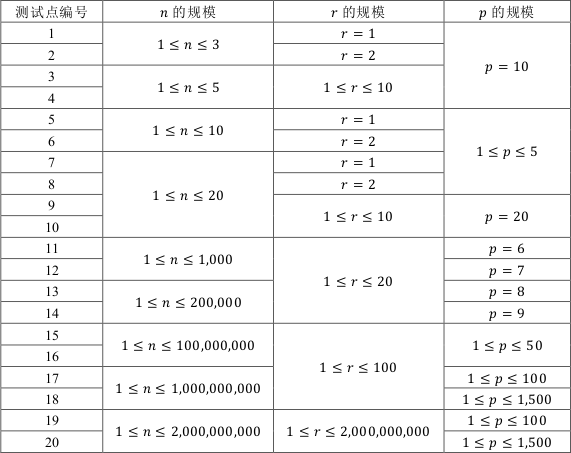# YZOJ P3098 [JLOI2016]成绩比较

• ### 题目描述

G系共有 $$n$$ 位同学，$$m$$ 门必修课。这 $$n$$ 位同学的编号为 $$0$$ 到 $$n-1$$ 的整数，其中B神的编号为 $$0$$ 号。

# YZOJ P3033 背包

• ### 题目描述

（即每种物品按照 $$1,2,3, \cdots ,b_i$$ 编号的方案数和不编号的方案数。）

• ### 数据规模与约定

Source: BZOJ 2658…

…

# YZOJ P2163 [THUSC2015]解密运算

• ### 数据规模与约定

$$N,M \leq 200000$$ 。

…

# YZOJ P3791 餐馆

• ### 数据规模与约定Source：ARC067 F – Yakiniku

# YZOJ P3846 [2018省队集训]Yist

• ### 题目描述

$$Alkri$$ 想在平面直角坐标系的第一象限中依次画 $$n$$ 个与两坐标轴均相切的圆，其中，第 $$1$$ 个圆的半径为 $$r$$，之后的每个圆都比上一个圆大，且与上一个圆相切，也就是说，对所有整数 $$2 \leq i \leq n$$，第 $$i$$ 个圆的半径大于第 $$i-1$$ 个圆的半径且与第 $$i-1$$个圆相切。• ### 数据规模与约定…